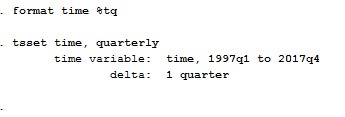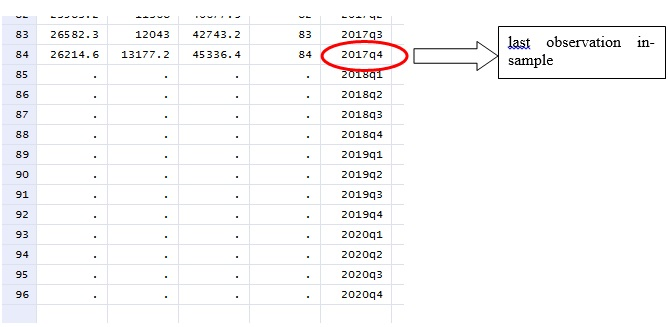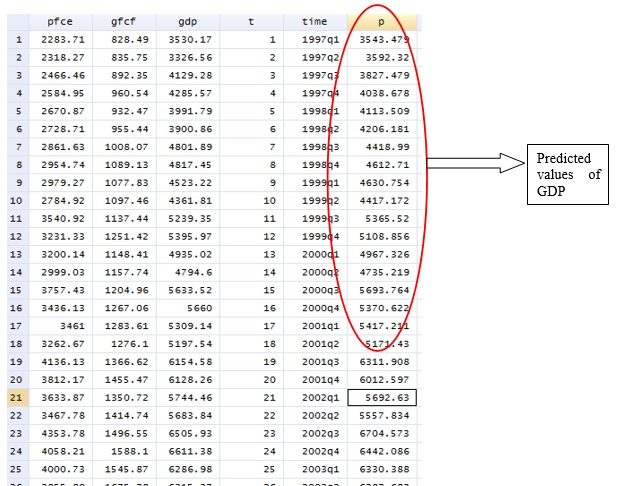# How to perform point forecasting in STATA?

A previous article demonstrated how to predict values for a variable that follows an autoregressive process. It showed that the first step is to identify an appropriate order of the autoregressive process. Then perform ARIMA modelling of the variable before generating the forecast. This article explains how to perform point forecasting in STATA, where one can generate forecast values even without performing ARIMA. Therefore, it is useful in any time series data.

Forecasting is an important part of time series analysis. A point forecast is a singular number which represents the estimate of the true but unknown value of a variable at a future date. The data on Gross Domestic Product (GDP), Gross Fixed Capital Formation (GFC) and Private Capital Consumption (PFC) for the same time period (1997 to 2017) as considered in the previous article is used.

## Performing point forecasting in STATA

### Step 1: Declare data as time series

The first step is to declare the data to be time series. For this, follow the below steps.

Stata command:This will arrange the time series data as follows.Figure 1: Quarterly time series data of variables for point forecasting in STATA

In the figure above, the last observation is 2017. To use point forecasting, extend the data for some more years depending on which forecast values are needed using ‘tsappend’ command.

```tsappend, add(3)
tsappend, last (2020Q4) tsfmt(tq)```

This command will add time period until 2020.Alternatively, use the following command.Figure 2: Extended time periods generated for point forecasting in STATA

Observations up to 2017 are ‘in-sample’ observations whereas observations after 2017 are ‘out of the sample’ observations (figure above).

### Step 2: Use regression coefficients

Then use the regression coefficients with the following command.

`regress gdp gfcf pfce`

After the regression, use the ‘predict’ command for point forecasting, so long as the regressors are available. ‘p’ is any new variable representing GDP (since GDP is the dependent variable in the regression model). Step 3: Use the ‘predict’ command.

`predict p`

In-sample and out of sample data: The command ‘predict p’ will generate forecast values for in sample observations and out-of-sample observations.

To restrict the forecasting to be in‐sample (for quarterly data), use the following command.

```predict p if t<=tq(2017q4)
predict yp if t>tq(2017q4)```

For the out of sample (for quarterly data) prediction use the following command. This command will give forecast values of gdp, (here in p variable) in the data set (figure below).Figure 3: Point predicted values of GDP for point forecasting in STATA

To plot GDP and ‘p’ in graph, use the following command.

`tsline gdp p`

The figure below will appear.Data plot of actual and forecasted values of GDP

The figure above represents the actual values and forecast values of GDP (p variable). The figure shows that there is a very slight difference actual GDP and forecast GDP values. It implies that in this case, point forecasting can effectively generate forecasted values of GDP for any time period in future.

## Shortcomings of point forecasting

In point forecasting, the prediction is possible at each specific point of the data series. However, one criticism of point forecasting is that it does not provide the degree of uncertainty that is associated with the forecast. To take into account variability in forecasting, interval forecasting is applicable as an alternative to point forecasting.

### Saptarshi Basu Roy Choudhury

Senior Research Analyst at Project Guru
Saptarshi has done his M. Phil in International Trade and Development and Masters in Economics from Jawaharlal Nehru University, New Delhi. His academic interests include issues related to economics of climate change, regulation and contemporary trade theories. He has a keen interest in current affairs and likes to read and travel in his spare time.

#### Latest posts by Saptarshi Basu Roy Choudhury (see all)We are looking for candidates who have completed their master's degree or Ph.D. Click here to know more about our vacancies.